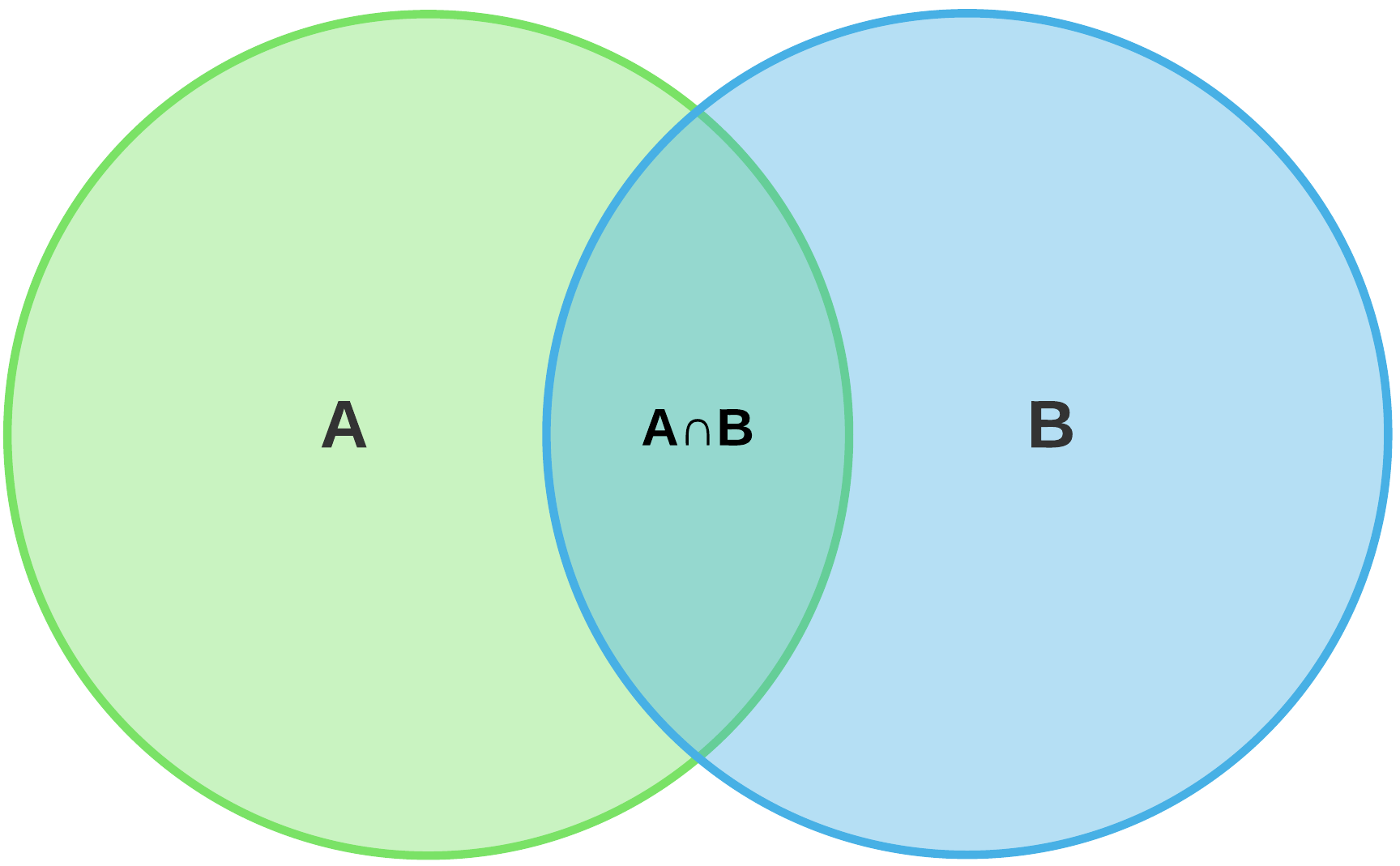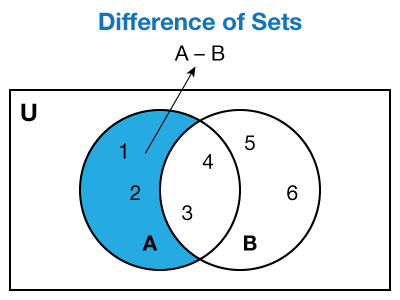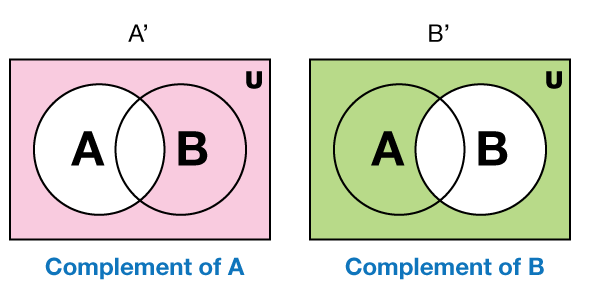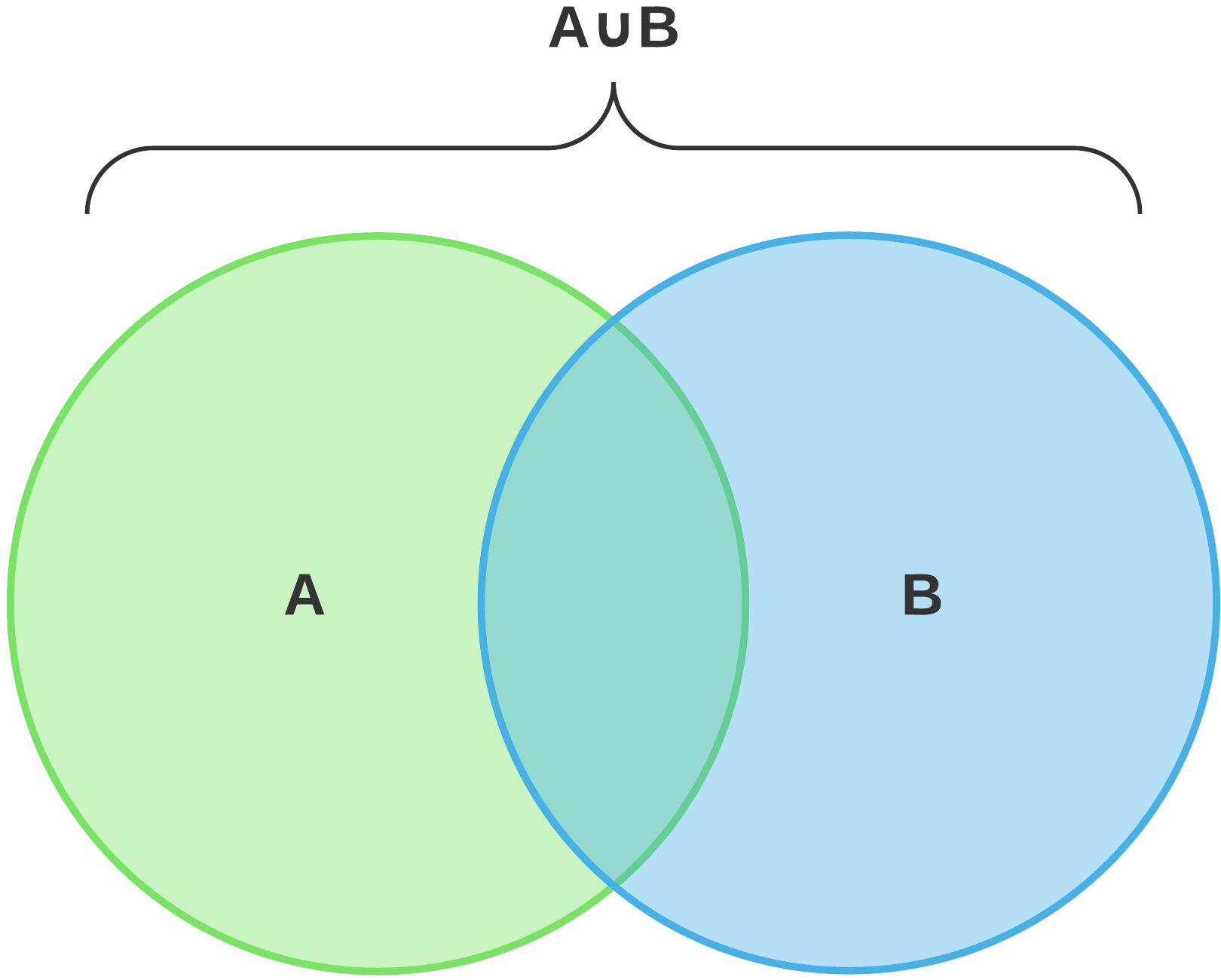# Operations on Sets

Go back to  'Sets'

## Introduction to Operations on Sets

The understanding and use of sets is one of the most unique aspects of mathematics. It helps us understand discrete numbers and objects and also helps to understand abstract math. A set can be a collection of like and unlike objects. For example, in your classroom, there are both girls and boys. You could have a set of the heights of all boys, a set of heights of all girls, or even a set of the heights of all students. You could have a set of all students who are taller than 5 feet and another set of all students whose heights are below 5 feet.

## The Big Idea: Operations on sets

### A simple idea: What does it mean to operate using sets?

Once you have decided on the criteria for deciding on the elements of a set, you can use these sets to visualize abstract concepts and operate on sets. The first thing to know and understand in set theory is the concept of a Venn diagram. A Venn diagram is defined as a diagram which represents a circle in which the elements of a set are enclosed, as per the defining criteria. The interesting thing about a Venn diagram is that it need not be always a collection of numbers. Like we said earlier, a Venn diagram can also be about abstract concepts. For starters, let us see how Venn diagrams are represented.## How is it important?

### What constitutes a set?

The objects inside a set are placed according to certain defining criteria. You could have a set of real numbers or a set of integers. You could have a set of odd numbers less than 100, or you could have a set of even numbers between 50 and 100.

Depending on what criteria you choose for creating a set, you will have zero, 1, 2 or more elements in a set. But the operations on sets are not the usual addition, subtraction, multiplication or division that we do with numbers. Let us see how we can operate sets.### Complement of a set

Before we go on to understand set operations, one important concept to understand is the complement of a set. A complement of a set consists of a set which includes all the elements which are not in that set. If we take the set mentioned above (set of odd numbers less than 30), then the complement of that set would be all the numbers in the number system outside these 15 numbers. If a set consists of the four members of your family, then the complement of that set would consist of all the people in the world except for these 4 people. If there is a set consisting of all left handed humans in the world, then the complement of this set would consist of all humans in the world who are right handed or ambidextrous.### Operations of sets

We will look at the union and intersection of sets. Union is represented by the symbol U, while intersection is represented by the symbol If there are two sets A and B, then the union and intersection are respectively represented by

Union of A and B  :  A U B
Intersection of A and B  :  A ∩ B

Union of two sets refers to the set containing elements belonging to either A or B. Intersection of two sets refers to the set containing elements belonging to both A and B.
To understand this concept better, let us consider the two finite sets A and B, consisting of the following elements:

A = (1, 2, 5, 8, 12, 15, 20)
B = (3, 5, 8, 10, 12, 15, 18, 25)

A U B = (1, 2, 3, 5, 8, 10, 12, 15, 18, 10, 25)
A ∩ B = (5, 8, 12, 15)

Did you notice what happened here? In the union, we mentioned only once all the elements which occurred in both sets, like 5, 8, 12, and 15. And in the intersection, the only elements that were included were the elements that occurred in both sets, like 5, 8, 12, and 15.

If you want to see the visual representation, you need to use Venn diagrams. This   gives you the opportunity of learning about complements, unions, and intersections by yourself.